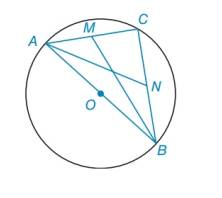Chapter 6.2, Problem 35E### Elementary Geometry for College St...

6th Edition
Daniel C. Alexander + 1 other
ISBN: 9781285195698

#### Solutions

Chapter
Section### Elementary Geometry for College St...

6th Edition
Daniel C. Alexander + 1 other
ISBN: 9781285195698
Textbook Problem
13 views

# Given: A B ¯ is a diameter of ⊙ ο M is the midpoint of chord A C ¯ N is the midpoint of chord C B ¯ M B = 73 ,     A N = 2 13 Find: The length of diameter A B ¯To determine

To find:

The length of diameter AB¯.

Explanation

Calculation:

Given,

First we have to find mc.

By the theorem, “An angle inscribed in a semicircle is a right angle”.

Since ABC is a semicircle, mc=90°

It is given that, M is a midpoint of AC¯.

Assume that, AM=MC=x.

Also it is given that, N is a midpoint of CB¯.

Then take, CN=NB=y.

Consider the right angle triangle ANC,

Now by Pythagorean theorem,

AC2+CM2=AN2

(AM+MC)2+CN2=AN2

(x+x)2+y2=(213)2

(2x)2+y2=4×13

4x2+y2=52 …(1)

Consider the right angle triangle MBC,

Again by Pythagorean theorem,

MC2+CB2=MB2

MC2+(CN+NB)2=MB2

x2+(y+y)2=(73)2

x2+4y2=73 …(2)

Solve the equations (1) and (2)

### Still sussing out bartleby?

Check out a sample textbook solution.

See a sample solution

#### The Solution to Your Study Problems

Bartleby provides explanations to thousands of textbook problems written by our experts, many with advanced degrees!

Get Started

#### Evaluate: a. 2332 b. [(13)3]1/3

Applied Calculus for the Managerial, Life, and Social Sciences: A Brief Approach

#### Graph the following functions. 11.

Mathematical Applications for the Management, Life, and Social Sciences

#### Using for |x| < 1,

Study Guide for Stewart's Multivariable Calculus, 8th